# Graphing ordered pairs and writing an equation from a table of values in context Online Quiz

#### Excel Pivot Tables and Dashboard. Simple & Quick tutorial!

Most Popular

7 Lectures 58 mins

#### Learn HTML Tables

15 Lectures 59 mins

#### Excel Analytics - Data Analysis with Pivot-Tables and Charts

46 Lectures 2.5 hours

Following quiz provides Multiple Choice Questions (MCQs) related to Graphing ordered pairs and writing an equation from a table of values in context. You will have to read all the given answers and click over the correct answer. If you are not sure about the answer then you can check the answer using Show Answer button. You can use Next Quiz button to check new set of questions in the quiz.Q 1 - Plot the ordered pairs described by the table. Write an equation relating x and y.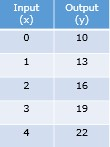### Explanation

Step 1:

The equation of the ordered pairs of given table is

y = 10 + 3x

Step 2:

The plot of the ordered pairs of given table −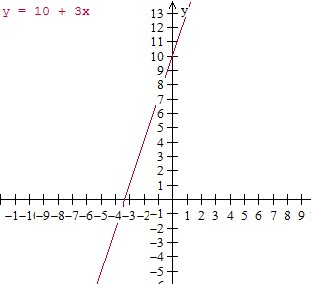Q 2 - Plot the ordered pairs described by the table. Write an equation relating x and y.### Explanation

Step 1:

The equation of the ordered pairs of given table is

y = 3 + 4x

Step 2:

The plot of the ordered pairs of given table −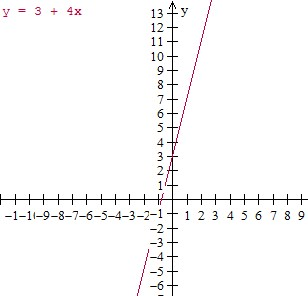Q 3 - Plot the ordered pairs described by the table. Write an equation relating x and y.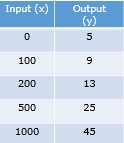### Explanation

Step 1:

The equation of the ordered pairs of given table is

y = 5 + $\frac{4x}{100}$

Step 2:

The plot of the ordered pairs of given table −Q 4 - Plot the ordered pairs described by the table. Write an equation relating x and y.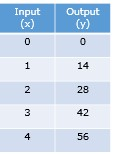### Explanation

Step 1:

The equation of the ordered pairs of given table is

y = 14x

Step 2:

The plot of the ordered pairs of given table −Q 5 - Plot the ordered pairs described by the table. Write an equation relating x and y.### Explanation

Step 1:

The equation of the ordered pairs of given table is

y = 12x

Step 2:

The plot of the ordered pairs of given table −Q 6 - Plot the ordered pairs described by the table. Write an equation relating x and y.### Explanation

Step 1:

The equation of the ordered pairs of given table is

y = 3x

Step 2:

The plot of the ordered pairs of given table −Q 7 - Plot the ordered pairs described by the table. Write an equation relating x and y.### Explanation

Step 1:

The equation of the ordered pairs of given table is

y = 4x

Step 2:

The plot of the ordered pairs of given table −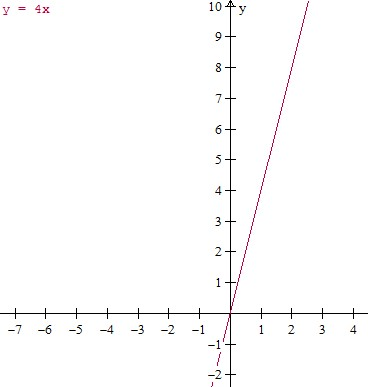Q 8 - Plot the ordered pairs described by the table. Write an equation relating x and y.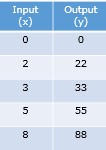### Explanation

Step 1:

The equation of the ordered pairs of given table is

y = 11x

Step 2:

The plot of the ordered pairs of given table −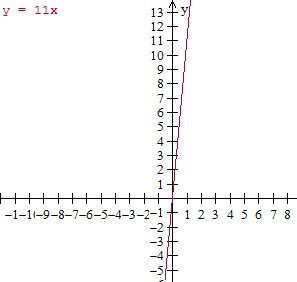Q 9 - Plot the ordered pairs described by the table. Write an equation relating x and y.### Explanation

Step 1:

The equation of the ordered pairs of given table is

y = 0.5 + $\frac{x}{4}$

Step 2:

The plot of the ordered pairs of given table −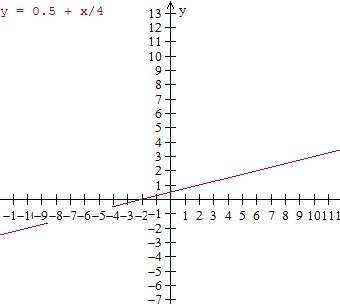Q 10 - Plot the ordered pairs described by the table. Write an equation relating x and y.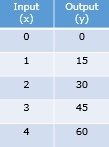### Explanation

Step 1:

The equation of the ordered pairs of given table is

y = 15x

Step 2:

The plot of the ordered pairs of given table −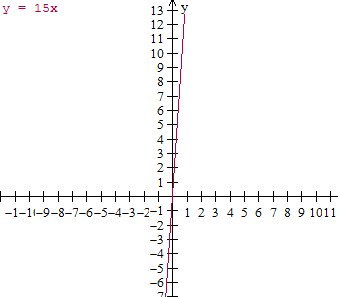graphing_ordered_pairs_and_writing_an_equation_from_table_of_values_in_context.htm